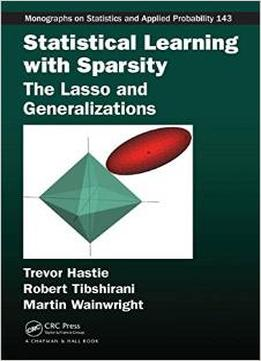# Statistical Learning With Sparsity: The Lasso And Generalizations by Robert Tibshirani / 2015 / English / PDF

Discover New Methods for Dealing with High-Dimensional Data A sparse statistical model has only a small number of nonzero parameters or weights; therefore, it is much easier to estimate and interpret than a dense model. Statistical Learning with Sparsity: The Lasso and Generalizations presents methods that exploit sparsity to help recover the underlying signal in a set of data. Top experts in this rapidly evolving field, the authors describe the lasso for linear regression and a simple coordinate descent algorithm for its computation. They discuss the application of ℓ1 penalties to generalized linear models and support vector machines, cover generalized penalties such as the elastic net and group lasso, and review numerical methods for optimization. They also present statistical inference methods for fitted (lasso) models, including the bootstrap, Bayesian methods, and recently developed approaches. In addition, the book examines matrix decomposition, sparse multivariate analysis, graphical models, and compressed sensing. It concludes with a survey of theoretical results for the lasso. In this age of big data, the number of features measured on a person or object can be large and might be larger than the number of observations. This book shows how the sparsity assumption allows us to tackle these problems and extract useful and reproducible patterns from big datasets. Data analysts, computer scientists, and theorists will appreciate this thorough and up-to-date treatment of sparse statistical modeling.

views: 566